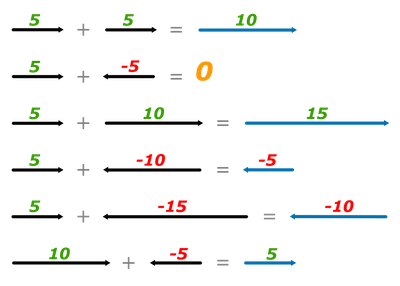# Scalar and Vector Quantities

Scalar and vector quantities are the two types of measurement tools in physics. . A quantity can be either scalar or vector. Consider a car going down the road at a speed of 50km/h and has a velocity of 50km/h in the northeast direction. Here we refer the speed as a scalar quantity and the velocity as a vector quantity.

### Scalar quantities

A scalar quantity has only magnitude and can be represented by the length of a straight line. Some examples are time, mass, temperature and speed.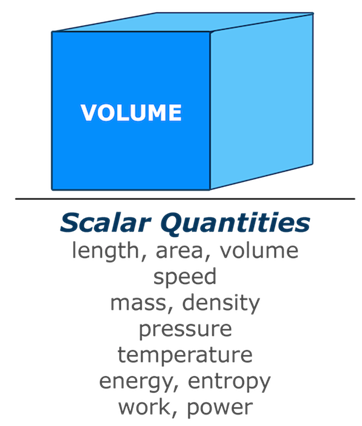In the following time scale figure, a time interval of 30 seconds is represented as a straight line with equal parts.

SCALAR QUANTITY TIME SCALE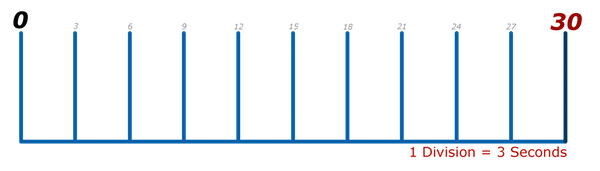### Vector quantities

A vector quantity has both magnitude and direction. When doing any mathematical operation on a vector quantity, you must consider both magnitude and the direction. Some examples are force, acceleration, lift, drag, thrust and velocity.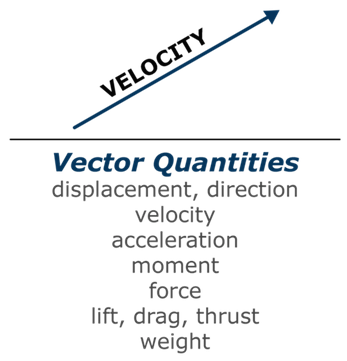Vector quantities are often graphically represented by placing a small arrow over the quantity, or by writing the quantity in a bold style. It is also common to indicate a vector by drawing an arrow whose length is proportional to the magnitude of the vector and whose direction specifies the vector orientation.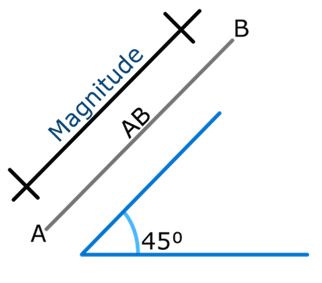Its magnitude (length) and direction (angle) are measured using the scale and the protractor, respectively. The resultant represents the single force that replaces the original force, with the same body effect.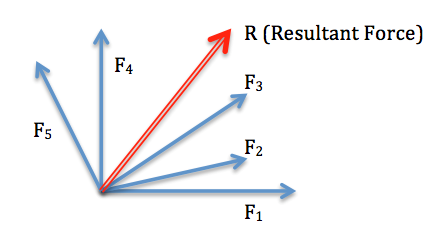In the following space diagram, a force of 5N is a vector quantity. In the vector line, the length represents magnitude and the angle represents direction, along with sense.

VECTOR QUANTITY SPACE DIAGRAM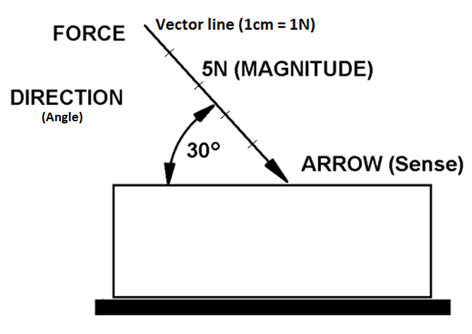Two vector quantities

In case of two vector quantities, accurate graphical representation helps in drawing conclusion. If two forces are applied to a body in any direction, draw a vector diagram with the data provided in order to find the force and direction of a body. Any scale can be used in the diagram. A resultant single force that can hold the original force is known as equilibrant. It has equal magnitude and opposite sense. The final vector diagram for two vector quantities should be in a closed triangle form known as triangle of forces.

Example

As shown in figure below, two forces are acting on a free moving body. Find in which direction and with what force the body will move.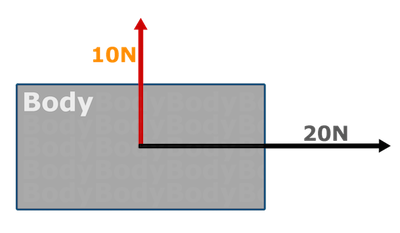To solve, first draw a vector diagram representing the forces. Start from any force and add the next vector diagram representing the force from where the first vector ended. (Scale: 1cm = 2N).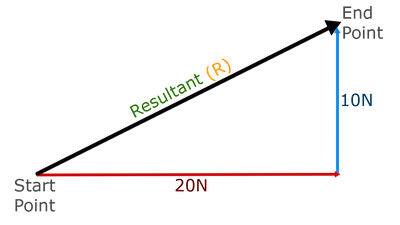The resultant force is equal to the distance represented by R (measured using drawing scale) and the direction represents the angle between the vector lines 20N and R (measured using protector).

Another way of finding the Resultant force is by using Pythagoras and its direction (angle) by using trigonometry.

More than two vector quantities

The same vector diagram rules are followed in case of handling more than two forces. It should be drawn accurately in the order provided.

Example

A body is subjected to the forces as shown in the space diagram below. Determine the direction in which the body likely to move.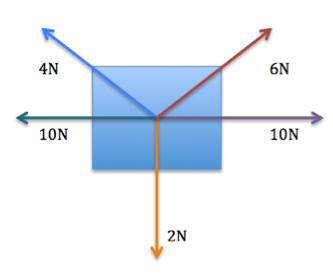Draw a vector diagram (Scale 1cm = 2N).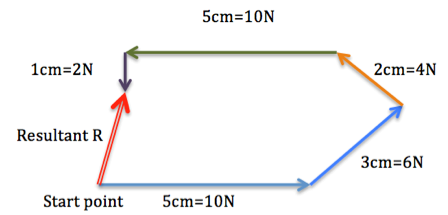• R = 2.3 cm x 2N = 4.6 N (distance measured using drawing scale)
• Angle a = 79o (Angle measured using protector)

Thus, the body will move with the force of 4.6N in the direction of W79N.